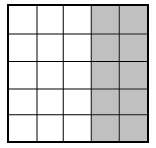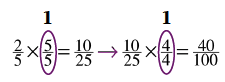Home > CC1MN > Chapter cc21 > Lesson cc21.2.5 > Problem1-103

1-103.In problem 1-95, Lila rewrote $\frac { 10 } { 25 }$ as $\frac { 40 } { 100 }$ and represented it with the picture at right. Tony thinks this fraction is also equal to $\frac { 2 } { 5 }$. Homework Help ✎

1. Is Tony correct? Use the picture or calculations to explain your reasoning. Write your answer in complete sentences.

Examine the picture and notice how the square is broken up into $25$ square units. If you created a $100$ square unit box and shaded in $40$ of the squares, would that be the same as having four of the above shapes placed together to form a box?

What about a rectangle with $5$ square units and $2$ shaded squares? Can the shape above be rearranged to form 5 rectangles like the one pictured at below?Each of the fractions are equivalent to each other because of the multiplicative identity as noted in the Math Notes box in this lesson.Consider why using the multiplicative identity changes the numbers of the fractions, but they are all still equivalent.

2. How could Tony write an equivalent (equal) fraction using tenths? That is, what fraction in the form $\frac { ? } { 10 }$ can represent the diagram above?

$\frac{2}{5}\rightarrow\frac{?}{10}$

If the equivalent fraction denominator desired is $10$, then the denominator of $5$ must be multiplied by what number?

Now, consider the multiplicative identity from part (a).
Now that you have solved for the denominator, what must the numerator be?

$\frac{4}{10}$